# Improving Physical Parametrizations in Climate Models using Machine Learning

Oct. 5, 2018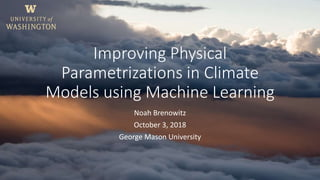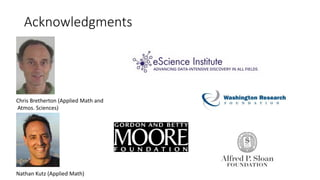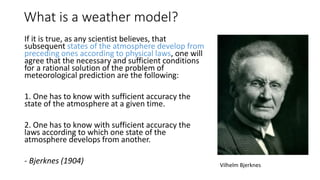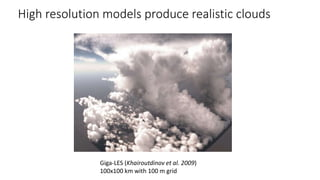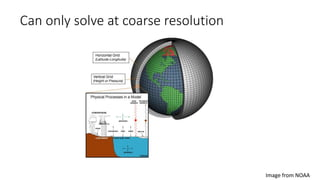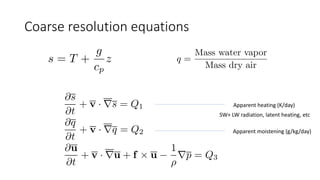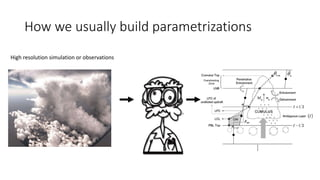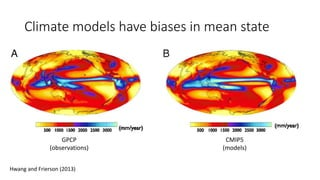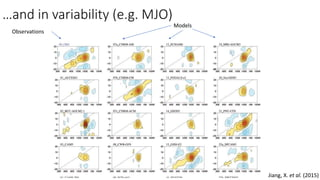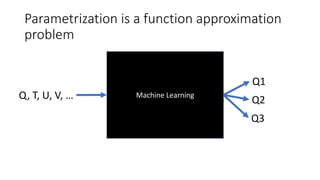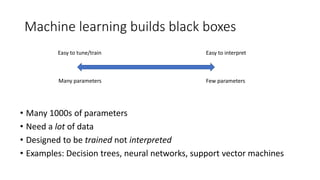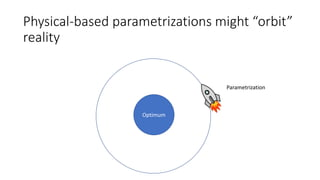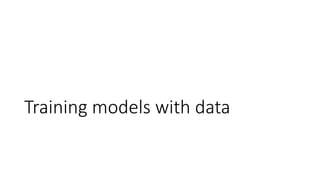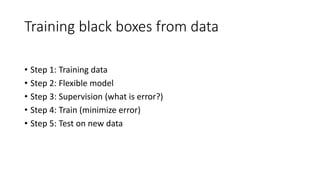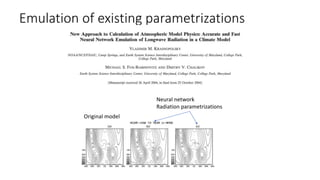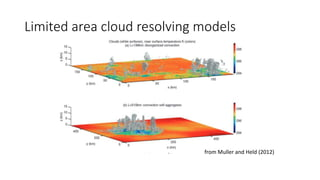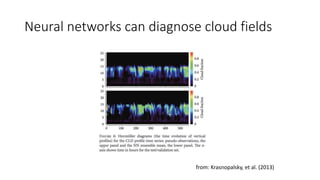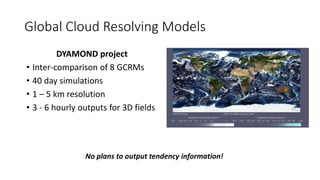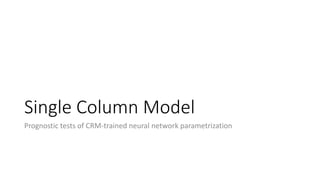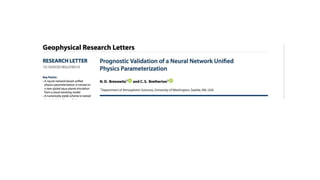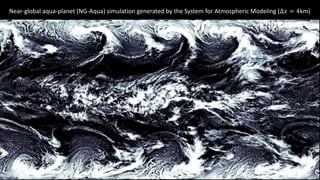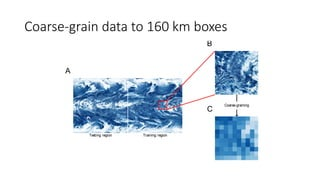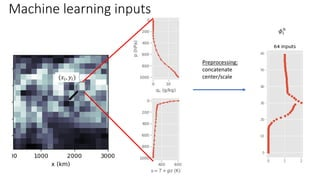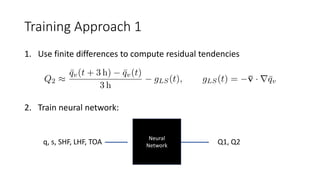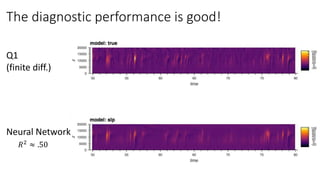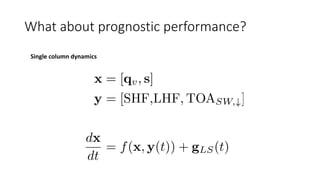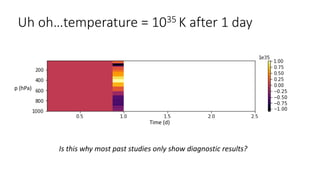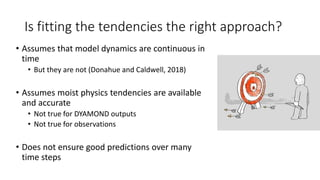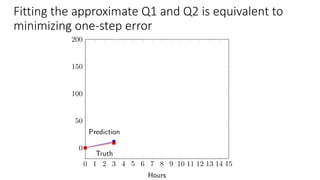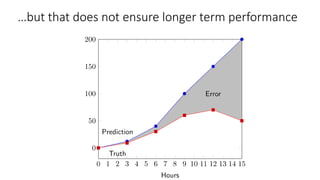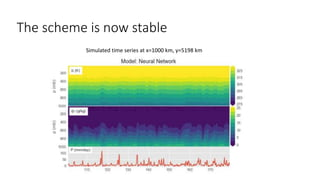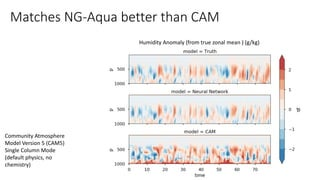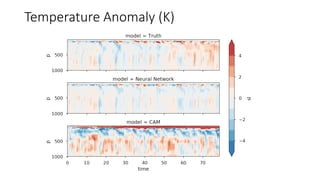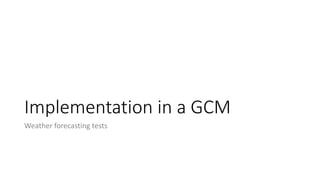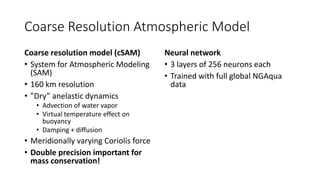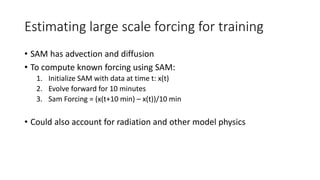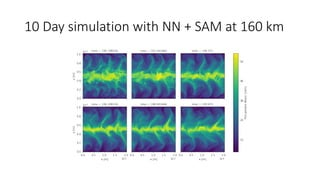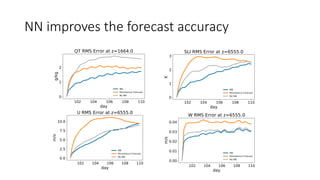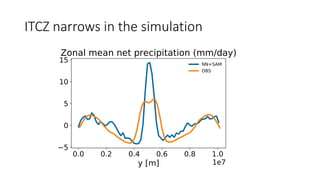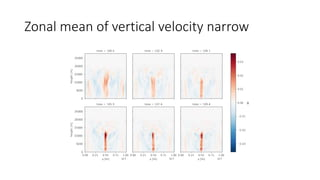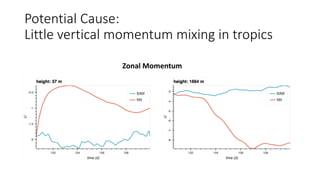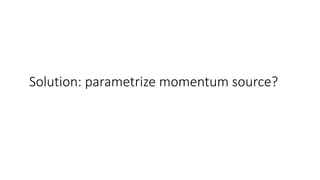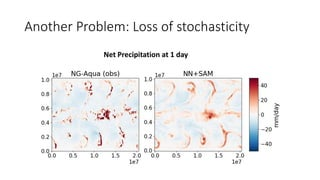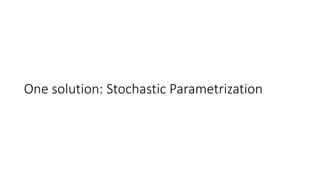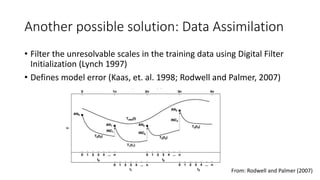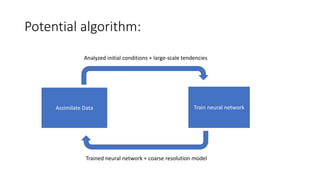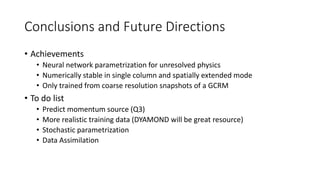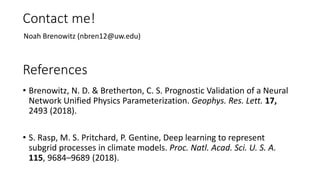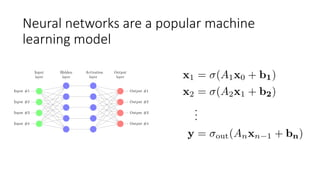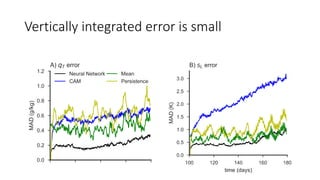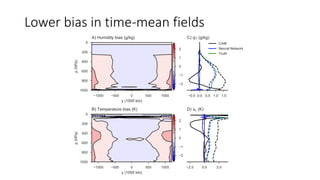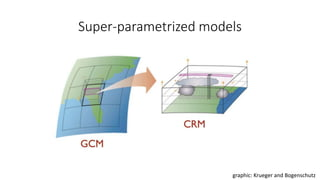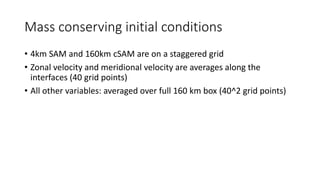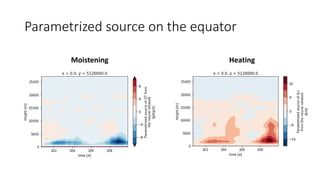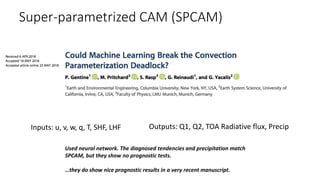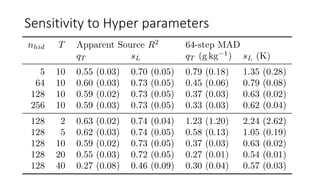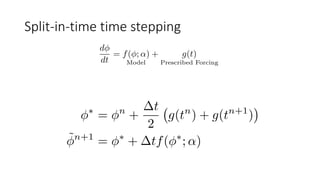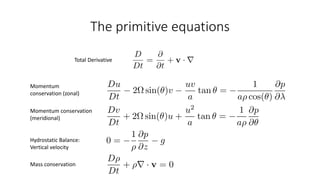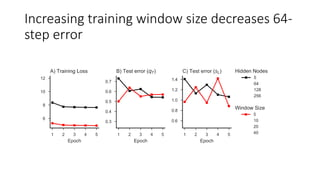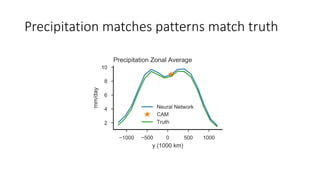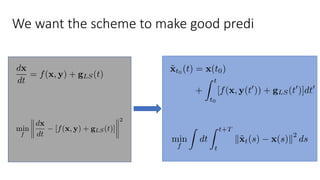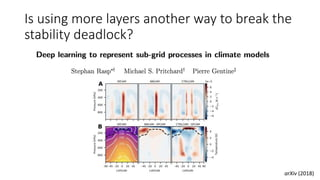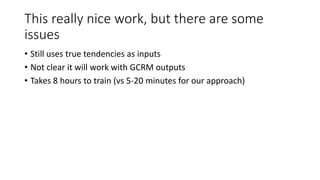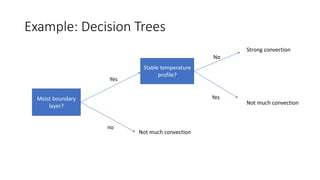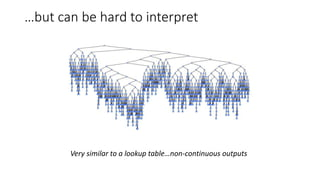1 of 67

### Improving Physical Parametrizations in Climate Models using Machine Learning

• 1. Improving Physical Parametrizations in Climate Models using Machine Learning Noah Brenowitz October 3, 2018 George Mason University
• 2. Acknowledgments Nathan Kutz (Applied Math) Chris Bretherton (Applied Math and Atmos. Sciences)
• 3. What is a weather model? If it is true, as any scientist believes, that subsequent states of the atmosphere develop from preceding ones according to physical laws, one will agree that the necessary and sufficient conditions for a rational solution of the problem of meteorological prediction are the following: 1. One has to know with sufficient accuracy the state of the atmosphere at a given time. 2. One has to know with sufficient accuracy the laws according to which one state of the atmosphere develops from another. - Bjerknes (1904) Vilhelm Bjerknes
• 4. High resolution models produce realistic clouds Giga-LES (Khairoutdinov et al. 2009) 100x100 km with 100 m grid
• 5. Can only solve at coarse resolution Image from NOAA
• 6. Coarse resolution equations Apparent heating (K/day) Apparent moistening (g/kg/day) SW+ LW radiation, latent heating, etc
• 7. How we usually build parametrizations High resolution simulation or observations
• 8. Climate models have biases in mean state CMIP5 (models) GPCP (observations) Hwang and Frierson (2013)
• 9. …and in variability (e.g. MJO) Observations Models Jiang, X. et al. (2015)
• 10. Parametrization is a function approximation problem Machine Learning Q1 Q2Q, T, U, V, … Q3
• 11. Machine learning builds black boxes • Many 1000s of parameters • Need a lot of data • Designed to be trained not interpreted • Examples: Decision trees, neural networks, support vector machines Easy to tune/train Easy to interpret Many parameters Few parameters
• 12. Optimum Parametrization Physical-based parametrizations might “orbit” reality
• 13. Training models with data
• 14. Training black boxes from data • Step 1: Training data • Step 2: Flexible model • Step 3: Supervision (what is error?) • Step 4: Train (minimize error) • Step 5: Test on new data
• 15. Emulation of existing parametrizations Original model Neural network Radiation parametrizations
• 16. Limited area cloud resolving models from Muller and Held (2012)
• 17. Neural networks can diagnose cloud fields from: Krasnopalsky, et al. (2013)
• 18. Global Cloud Resolving Models DYAMOND project • Inter-comparison of 8 GCRMs • 40 day simulations • 1 – 5 km resolution • 3 - 6 hourly outputs for 3D fields No plans to output tendency information!
• 19. But no previous prognostic tests with CRM data
• 20. Single Column Model Prognostic tests of CRM-trained neural network parametrization
• 22. Near-global aqua-planet (NG-Aqua) simulation generated by the System for Atmospheric Modeling (Δ𝑥 = 4km)
• 23. Coarse-grain data to 160 km boxes Training regionTesting region Coarse-graining A B C
• 24. Machine learning inputs 𝜙𝑖 𝑛 Preprocessing: concatenate center/scale(𝑥𝑖, 𝑦𝑖)
• 25. Training Approach 1 1. Use finite differences to compute residual tendencies 2. Train neural network: q, s, SHF, LHF, TOA Q1, Q2 Neural Network
• 26. The diagnostic performance is good! Neural Network Q1 (finite diff.) 𝑅2 ≈ .50
• 27. What about prognostic performance? Single column dynamics
• 28. Uh oh…temperature = 1035 K after 1 day Time (d) p (hPa) Is this why most past studies only show diagnostic results?
• 29. Is fitting the tendencies the right approach? • Assumes that model dynamics are continuous in time • But they are not (Donahue and Caldwell, 2018) • Assumes moist physics tendencies are available and accurate • Not true for DYAMOND outputs • Not true for observations • Does not ensure good predictions over many time steps
• 30. Fitting the approximate Q1 and Q2 is equivalent to minimizing one-step error
• 31. …but that does not ensure longer term performance
• 32. The scheme is now stable Simulated time series at x=1000 km, y=5198 km
• 33. Matches NG-Aqua better than CAM Community Atmosphere Model Version 5 (CAM5) Single Column Mode (default physics, no chemistry) Humidity Anomaly (from true zonal mean ) (g/kg)
• 34. Temperature Anomaly (K)
• 35. Implementation in a GCM Weather forecasting tests
• 36. Coarse Resolution Atmospheric Model Coarse resolution model (cSAM) • System for Atmospheric Modeling (SAM) • 160 km resolution • ”Dry” anelastic dynamics • Advection of water vapor • Virtual temperature effect on buoyancy • Damping + diffusion • Meridionally varying Coriolis force • Double precision important for mass conservation! Neural network • 3 layers of 256 neurons each • Trained with full global NGAqua data
• 37. Estimating large scale forcing for training • SAM has advection and diffusion • To compute known forcing using SAM: 1. Initialize SAM with data at time t: x(t) 2. Evolve forward for 10 minutes 3. Sam Forcing = (x(t+10 min) – x(t))/10 min • Could also account for radiation and other model physics
• 38. 10 Day simulation with NN + SAM at 160 km
• 39. NN improves the forecast accuracy
• 40. ITCZ narrows in the simulation
• 41. Zonal mean of vertical velocity narrow
• 42. Potential Cause: Little vertical momentum mixing in tropics Zonal Momentum
• 43. Solution: parametrize momentum source?
• 44. Another Problem: Loss of stochasticity Net Precipitation at 1 day
• 45. One solution: Stochastic Parametrization
• 46. Another possible solution: Data Assimilation • Filter the unresolvable scales in the training data using Digital Filter Initialization (Lynch 1997) • Defines model error (Kaas, et. al. 1998; Rodwell and Palmer, 2007) From: Rodwell and Palmer (2007)
• 47. Potential algorithm: Assimilate Data Train neural network Trained neural network + coarse resolution model Analyzed initial conditions + large-scale tendencies
• 48. Conclusions and Future Directions • Achievements • Neural network parametrization for unresolved physics • Numerically stable in single column and spatially extended mode • Only trained from coarse resolution snapshots of a GCRM • To do list • Predict momentum source (Q3) • More realistic training data (DYAMOND will be great resource) • Stochastic parametrization • Data Assimilation
• 49. References • Brenowitz, N. D. & Bretherton, C. S. Prognostic Validation of a Neural Network Unified Physics Parameterization. Geophys. Res. Lett. 17, 2493 (2018). • S. Rasp, M. S. Pritchard, P. Gentine, Deep learning to represent subgrid processes in climate models. Proc. Natl. Acad. Sci. U. S. A. 115, 9684–9689 (2018). Noah Brenowitz (nbren12@uw.edu) Contact me!
• 50. Neural networks are a popular machine learning model
• 51. Sometimes negative
• 52. Vertically integrated error is small
• 53. Lower bias in time-mean fields
• 54. Super-parametrized models graphic: Krueger and Bogenschutz
• 55. Mass conserving initial conditions • 4km SAM and 160km cSAM are on a staggered grid • Zonal velocity and meridional velocity are averages along the interfaces (40 grid points) • All other variables: averaged over full 160 km box (40^2 grid points)
• 56. Parametrized source on the equator Moistening Heating
• 57. Super-parametrized CAM (SPCAM) Inputs: u, v, w, q, T, SHF, LHF Outputs: Q1, Q2, TOA Radiative flux, Precip Used neural network. The diagnosed tendencies and precipitation match SPCAM, but they show no prognostic tests. …they do show nice prognostic results in a very recent manuscript.
• 58. Sensitivity to Hyper parameters
• 59. Split-in-time time stepping
• 60. The primitive equations Total Derivative Momentum conservation (zonal) Momentum conservation (meridional) Hydrostatic Balance: Vertical velocity Mass conservation
• 61. Increasing training window size decreases 64- step error
• 62. Precipitation matches patterns match truth
• 63. We want the scheme to make good predi
• 64. Is using more layers another way to break the stability deadlock? arXiv (2018)
• 65. This really nice work, but there are some issues • Still uses true tendencies as inputs • Not clear it will work with GCRM outputs • Takes 8 hours to train (vs 5-20 minutes for our approach)
• 66. Example: Decision Trees Moist boundary layer? no Yes Stable temperature profile? Yes No Strong convection Not much convection Not much convection
• 67. …but can be hard to interpret Very similar to a lookup table…non-continuous outputs

### Editor's Notes

1. Videos available here: https://cimss.ssec.wisc.edu/data/
2. I’d first like to acknowledge my co-mentor Nathan Kutz, and the following organizations for funding my work
3. But what is a weather/climate model? The father of meteorology Vilhem Bjerknes said this quote...which I will read <read slide> In this talk I will focus on number 2 <click> Traditionally, data has had it’s strongest impact on point 1, but in this work we focus on using data in part 2.
4. Now, on certain scales, we know the physical laws describing atmospheric motions well enough to create extremely realistic depictions of clouds and other small-scale atmospheric phenenomena. This is a visualization from a large-eddy-simulation performed using the SAM model on a 100m grid.
5. Now, this simulations uses a similar number of degrees of freedom for one 100 km squared box as a climate model does for the whole planet. So it’s just not practical to perform simulations at that level of accuracy.
6. Instead of solving the fine resolution equations we end up solving the coarse-resolution equivalent. Here, I am showing the coarse resolution budgets of dry static energy, water vapor mixing ratio, and momentum. These budgets are forced by some terms which are known on the coarse-grid scale, such as advection, and the coriolis force. Bar denotes the average over a GCM grid box. The grad bar is a coarse grained approximation of the gradient. The right hand side denotes the residual terms, which are known as the apparent heating (Q1), which includes raditiona nad latent heating, and the apparent moistening (Q2), which describes phase changes of water. Likewise there is a residual term in the momentum budget (Q3) related to turbulent mixing. The taks of parametriztion is to close these budgets, and write Q1-Q3 as a functions of the coarse-resolution variables alone. &\frac{\partial \overline{s}} {\partial t} + \mathbf{\overline{v}} \cdot \overline{\nabla} \overline{s} = Q_1 \\   &\frac{\partial \overline{q}} {\partial t} + \mathbf{\overline{v}} \cdot \overline{\nabla} \overline{q} = Q_2\\  &\frac{\partial \overline{\mathbf u}} {\partial t} + \mathbf{\overline{v}} \cdot \overline{\nabla} \overline{\mathbf u} + \mathbf{f}\times \overline{\mathbf{u}}  -\frac{1}{\rho} \nabla \overline{p} = Q_3\\
7. So how do we normally go about this. A scientist will interpret a complex set of simulations or observatiosn and reduce them to a simplified flow chart. This flow chart will then be turned into a Fortran code, and if it’s succesful be intergrated into a climate model. While this approach has yielded great benefits, it is evident that it can improved. For example, the pace of development in parametrization is very slow. In particular, deep convective parameterizations have not changed much in the past two decades even though many new high resolution models and observations have become available. I will argue that the main bottleneck process is this person…the scientist.
8. Perhaps as a result, many climate models have large biases in the mean state. Here I am showing a plot of the famous double-ITCZ bias, which is a precipitation bias in the tropics. On the left, are observations, and on the rightn is the multi model mean of many climate models.
9. There are also problems in the variability. In particular, models struggle to simulate the main intraseasonal oscillation in the tropics, the Madden Julian Oscillation. Each panel of this plot depicts lag regression diagrams of the rainfall averaged between 10S and 10N. One of the MJO’s most basic features is its eastward propagation. Unfortunately, many models can not replicate this.
10. So how do we move past this situation? Essentially, param is a function approximation problem. It takes in the profiles of humiidity, temperature, etc and produces the apparent source terms Q1, Q2, and Q3. By using simplified physical models, we essentially limit the class of functions that we can use to just those which are interpretable by humans at the cost of accuracy. Here, I will argue that treating parametrizations as a black box machine learning problem can help us move past this bottleneck. While many may not like black boxes, the fact is many or most physically based parametrizations already are black boxes to most climate modelers. This is even more true when considering the fully coupled interactions of many different parametrizations.
11. The defining attribute of machine learning is the complexity of the models it uses. To illustrate this point, I would like to use this complexity spectrum. On the right are models, like most current parametrizations, that are easy to interpret because they have few parameters. On the other side, are models that have many thousands of parameters, which are easier to train. This might seam paradoxical, but in a higher dimensional parameter space there might be many more ”right answers” than in a low-dimensional space. Some examples include…<read slides>
12. Here is toy example of how a excessively low dimensional parameter space can orbit a optimal solution. Let this space ship be a typical phsyics parametrization, and let our task be to land at the optimum. If the space ship only controls in the azimuthal angle theta, then it will only ever orbit the optimum. To make it land, it will need at least one more parameter (the radius).
13. I’ve talked about the models a little a bit, so let’s talk about the training datasets. And this section will serve as an introduction to some past work on machine learning parametrization.
14. So how do we train a machine learning model with many 1000s of parameters? The key is to base everything off of a suitable training dataset.
15. The simplest possible training dataset, is the output of a known determinsitic function. This is known as emulation. Indeed, Krasnopalksy trained a simple neural network model to reprodcue the output of the community atmosphere models radiation code.
16. Another more interesting datasets, are simulations which explcitily resolve atmospheric convection, by having grid sizes less than 4 km or so. Because of their computational expense, these are usually run in limited area domains. One issue with this data, is that the tendencies we aim to estimate (e.g. Q1 and Q2 and Q3) are not outputs of the model, and have to be estimated.
17. Kransopalsky trained a neural network to reproduce the mean effect of clouds in a CRM simulation, and shoed some interesting diagnostic results. In other words, given only the humidity and temperature profiles, they could predict the instantaneous amount of precipitation and other fields like cloud fraction.
18. In my view, the most exciting datasets that we can use for machine learning are from global cloud resolving models. One important thing to note, is that there are no plans to output tendency information.
19. Unfortunately, unlike their emulation work, they and no others until very recently tested their methods prognostically.
20. In this section, I will discuss some work we recently published which prognostically tests a NN parametrization in a single column setting.
21. We published this work recently in GRL.
22. Our training dataset is a simplified GCRM simulation performed using the System for Atmospheric modeling (by Marat Khairtoudinov). We call it the near-global aquaplanet simulation or NGAqua Mention the aspects of the simulation Diurnal cycle mid latitude cyclones Tropics (w/ eastward disturbance) Now, this dataset was not designed for machine learning, so they did not store moisture or temperature budget information. And the sampling interval is relatively coarse in time.
23. For this study, we focused on the tropics, where moist atmospheric convection plays an especially important role. We coarse grain the 4 km NGAqua data onto a 160km grid, representative of a typical climate model resolution. This resolution was partially chosen so that the precipitation de-correlates over a time scale of about 3-6 hours.
24. Within each grid box we have measurements at different heights of humidity and dry static energy. There are 34 vertical grid points for each variable, which we concatenate into a single 68 dimensional vector. The data are normalized by subtracting the mean and dividing by a mass-weighted variance.
25. So as I said before, we did not have the moist physics tendencies available so the first approach we took was to estimate them using finite differences in time. And then train a neural network which depends on the humidity, static energy, surface fluxes, and TOA flux to preidct these approximate tendencies. Eq 1 Q_2 \approx \frac{\bar{q}_v(t+ \SI{3}{\hour})- \bar{q}_v(t)}{\SI{3}{\hour}} - g_{LS}(t),\qquad g_{LS}(t) = -\mathbf{\bar{v}} \cdot \nabla \bar{q}_v Eq 2 f^* = \argmin_f \left|| f([\mathbf{q}_v, \mathbf{s}, \text{LHF}, \text{SHF}, \text{TOA}_{SW,\downarrow}]) - [Q_1, Q_2] \right||^2
26. We find that when we train… we got good diagnostic performance. In this top panel I show the approximate apparent heating rates for a given location in the equator. The bottom shows the heating rates predicted by the neural network. The timing and magnitude of the strong heating events (which are associated with heavy rainfall and atmospheric convectoin) are quite similar.
27. So what about prognostic performance. We test this in the single column model framework. So first a bit of notiation….x are the prognostic variables which will be evolved forward in time. Y are the auxiliary inputs which we prescribe. Likewise, gLS(t) are the coarse-grid advection tendencies which we compute offline using centered differences. Then, the single column dynamics, treat g_LS and y as prescribed forcings which vary in time, but do not respond to the local fluctuations of humidity and temperature. This provides a simple prognostic testing framework, that can be simulate cheaply. \mathbf{x} &= [\mathbf{q}_v, \mathbf{s}] \\ \mathbf{y} &= [\text{SHF,LHF}, \text{TOA}_{SW,\downarrow}]\\ \\ \frac{d \mathbf{x}}{dt} &= f(\mathbf{x},\mathbf{y}(t)) + \mathbf{g}_{LS}(t)
28. However, when we run the model forward for many time steps we get dramatically unrealistic results.
29. First problem, we know model dynamics are not continuous. Changing the order of physical paramtrizations in a GCM can dramatically alter results.
30. As it turns out, approximating Q1 and Q2 using finite differences over three hours is equivalent to minimizing the one-step error made by the SCM.
31. …but that does not ensure longer term performance. To fix this, we instead minimize the accumulated error, over multiple time steps.
32. And we train the model in this way, we obtain a stable scheme.
33. Moreover, this scheme matches the NG-Aqua data much better than the single column version of CAM does. Here are moisture anomalies…
34. And temperature ones…
35. The next logical step is to couple this neural network to large-scale dynamics. In other words, put the neural network in a coarse resolution model.
36. To do this, we run the CRM we used to generate the NGAqua dataset, at the coarse-graining resolution of 160km. We chose to use this model, rather than a more established GCM like CAM, because the NGAqua data was not generated by a primitive equation model, and did not have spherical geometry. Perhaps, the most challenging aspect of this work I am about to present has been developing coarse resolution capabilities for the SAM mode. This includes adding hyperdiffusion, reading initial conditions from a netCDF. For instance, we found that coarse res SAM does not conserve mass when compiled in single precision. Another difficulty is that the neural network code is in python, but the model is in Fortran. So I have had to develop a interface layer for calling python from within fortran. On the other hand, the neural network training is pretty similar. We use a slightly deeper neural network with 3 layers. It was not much difficult to expand the traiing region outside of the tropics.
37. We also changed how we estimated the resolved/known “large-scale” forcing. Before, I just computed the advective derivative with centered differences, but now I actually use SAM to compute this. This procedure could also account for radiation and other model physics.
38. After training the model with these large-scale forcings, we then ran a simulation in coarse resolution SAM with the neural network on. Here are 6 snapshots of the precipitable water field through the course of this simulation. The hope is that these snapshots replicate the original NGAqua simulation very closely.
39. And when compute quantitative notions of forecast error, this seems to be the case. Here I have plotted the domain RMS forecast errors for 4 atmospheric variables. The blue curve is the simulation with the NN. The gray curve is a dry-dynamics only simulation, and the orange curve is a persistence forecast. At certain heights, the NN significantly improves the accruacy for the humidity and temperature. It struggles with the horizontal winds, and does an especially poor job with the vertical winds.
40. One big issue, that was visible a couple slides ago is that the ITCZ narrows dramatically in the NN+SAM simulation. Here, I plot the zonal average of net precipitaion in the final forecast day of the NN simulation compared with the all-time mean from NGAqua. The preicpitation is much more peaked near the “equator” of the model.
41. This can also be seen by looking at zonal average vertical motion in the tropics.
42. Since we do not predict the source of momentum, and SAM does not have a cumulus momentum transport or boundary layer scheme, we think that these problems might not be caused by problems with the NNs prediction of temperature and humidity forcing. In particular, it appears that there is not enough momentum mixing in the tropical lower atmosphere. For instance the easterlies in above the equator are too weak near the surface, but too strong aloft. Conceivably, this could alter the lower level convergence in the tropical regions.
43. An obvious solution is to parametrize CMT either using an off the shelf scheme or a neural network.
44. Another problem is a loss of stochasticity compared to the training data. This is clear by comparing the net precipitation after 1 day between NGAqua an the NN+SAM simulation. The NG simulation is much more speckled and has much higher peak rain rates.
45. One obvious solution is to make the output of the parametrization stochastic. We are starting up some collaborations to do this.
46. Another possible solution is to make the training data itself less stochatic, by using data assimilation to filter the ”unresolvable” scales. Indeed, some studies already discuss using data assimilation to define model error
47. This hints at a potential algorithm…. This also provides a loose coupling between the neural network training process and the large-scale dynamics. This provides a path towards using observations!
48. Now, I have always found that description a little confusing, so here are the equations. The values at each layer are just a linear transform of the previous layer followed by applying a nonlinear function. This nonlinear function is known as an activation function. So the parameters of a neural net are the weight matrices A_1,… and the constant vectors b_1…
49. Also the bias is much smaller with the neural network.
50. For example, the model needs to be initialized with winds that obey mass conservation.
51. We trained a neural network with these new large-scale forcings and ran an
52. More recently, Pierre Gentine, Mike Pritchard, and co authors.
53. Increasing nhid tends to capture the short term errors. Increasing nhid has much more effect on the 64-step errors. Increasing T helps is necessary for longer time accuracy. But does not hurt the short-term performance very much.
54. Our results are actually pretty sensitive to the type of time-stepping we use to advance the ODE forward in time. We find the best results when using split-in-time time stepping. \phi^* &= \phi^{n} + \frac{\Delta t}{2} \left(g(t^n) + g(t^{n+1})\right)\\ \tilde{\phi}^{n+1} &= \phi^* + \Delta t f(\phi^*;\alpha)
55. The atmosphere is goverened (at least in principle) by the so-called primitive equations There are three equations for the 3 componetns of velocity. These equations have acceleration terms. (click) Corilios terms becauset he erath rotates, and these terms because the earth is a sphere. The atmosphere is very thin, so the Dw/Dt terms in the vertical advection equation dissapear. It also has a mass conservation equation.
56. I don’t show T=2 because it is numerically unstable as shown before.
57. The solution we settled upon was to make good predictions over many time steps \min_f \norm{\frac{d \mathbf{x}}{dt} - [f(\mathbf{x},\mathbf{y}) + \mathbf{g}_{LS}(t)]}^2 \tilde{\mathbf{x}}_{t_0}(t) &=  \mathbf x(t_0) \\          &+ \int_{t_0}^t [f(\mathbf{x}, \mathbf y(t')) + \mathbf{g}_{LS}(t')] dt’ \min_f \int dt \int_{t}^{t + T} \norm{ \tilde{\mathbf x}_{t}(s) - \mathbf x(s)}^2 ds
58. One classic machine model is a decision tree, such as the one pictured here. Read the decision tree.
59. But they can be hard to interpret for many input variables. Also, they do not have continuous outputs, which seems a poor match for physics problems like convective parameterization.